# Test: Electromagnetic Fields Theory- 1

## 20 Questions MCQ Test Mock Test Series for SSC JE Electrical Engineering | Test: Electromagnetic Fields Theory- 1

Description
Attempt Test: Electromagnetic Fields Theory- 1 | 20 questions in 12 minutes | Mock test for SSC preparation | Free important questions MCQ to study Mock Test Series for SSC JE Electrical Engineering for SSC Exam | Download free PDF with solutions
QUESTION: 1

### A perfect magnetic conductor is defined to be a material for which...

Solution:

In a perfect magnetic conductor the value of has infinite magnetic conductivity (inverse of magnetic reluctance). In a perfect magnetic conductor the normal electric field and tangential magnetic field is zero while tangential electric field and normal magnetic field is equal.

QUESTION: 2

### As per Ohm’s law of magnetic circuit:

Solution:

Ohm’s law in case of magnetic circuits can be stated as, the magnetic flux developed in the circuit is directly proportional to magnetomotive force. The relation is similar to Ohm’s law and can be formed by replacing electro motive force (emf) with mmf and electric current (i) with magnetic flux.

Flux = mmf/reluctance.

QUESTION: 3

### For making a capacitor, it is better to select a dielectric having

Solution:

In a capacitor the value of capacitor is given by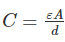hence with dielectric having high permittivity higher values of capacitance can be achieved with small capacitor size.

QUESTION: 4

Determine the reluctance (in Amp-turns/Wb) of a coil, when the flux through the coil is 25 Wb and the value of produced mmf is 50 Amp-turns

Solution:

Given that, mmf = 50 Amp-turns

Magnetic flux = 25 Wb

Reluctance = mmf/flux = 50/25 = 2 Amp-turns/Wb

QUESTION: 5

Determine the current (in A) through a 60 cm long solenoid when the solenoid has 400 turns and the value of magnetic field at the centre of the solenoid is 6 mT.

Solution: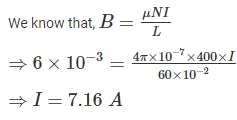QUESTION: 6

Permeance is analogous to:

Solution:

Permeance is a magnetic property, it is analogous to conductance. Reluctance is analogous to resistance.

QUESTION: 7

When a dielectric is placed in an electric field, the field strength _____.

Solution:

A dielectric is an electrical insulator that can be polarized by an applied electric field. When a dielectric is placed in an electric field, electric charges do not flow through the material as they do in an electrical conductor. Hence the electrical field strength decreases.

QUESTION: 8

Which one of the following materials cannot be used for permanent magnets?

Solution:

Of the given material, only iron cobalt alloy cannot be used to make permanent magnets.

QUESTION: 9

A conductor of length 0.6 m is situated at the right angle to a uniform magnetic field of flux density 4 Wb/sq. m. The conductor is moving with a velocity of 50 m/s. Calculate the emf induced (in V) n the conductor.

Solution:

Given that, Magnetic flux density (B) = 4 Wb/sq. m.

Length (l) = 0.6 m

Velocity (v) = 50 m/s

Emf induced is, E=Blvsinθ

E=4×0.6×50×sin90=120

QUESTION: 10

The graph shown below represents the B-H curve for _______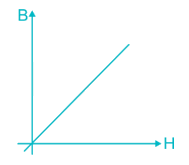Solution:

B-H curve is used to show the relationship between magnetic flux density (B) and magnetic field strength (H) for a particular material.

There is no hysteresis loss in air as air does not consists of magnetic material. So the B-H curve in the air should be a straight line.

According to the equation B = μH, this straight line passes through origin and has a slope of μ.

QUESTION: 11

If the magnetic susceptibility of any material is less than zero then the material is ________.

Solution:

In paramagnetic materials, the magnetic field in the material is strengthened by the induced magnetization. Hence the magnetic susceptibility value is a small positive.

In diamagnetic materials, the magnetic field in the material is weakened by the induced magnetization. Hence the magnetic susceptibility value is a small negative.

Ferromagnetic, ferrimagnetic materials possess permanent magnetization even without external magnetic field. Hence these materials have large positive value of magnetic susceptibility.

QUESTION: 12

Which one of the following is the CORRECT expression for magnetic susceptibility?

Solution:

Magnetic susceptibility is a dimensionless proportionality constant that indicates the degree of magnetization of a material in response to an applied magnetic field.

Magnetic susceptibility (X) of a material is the ratio of magnetization I (magnetic moment per unit volume) to the applied magnetizing field intensity H.

X = I/H

QUESTION: 13

What will be the voltage (in V) across a 8 H inductor, when the rate of change of current in the inductor is 0.5 A/sec.

Solution:

Given that, inductance (L) = 8 H

Rate of change of current = 0.5 A/sec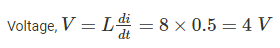QUESTION: 14

Determine the intensity of magnetization (in A/m) of a magnet when the pole strength of the magnet is 30 A-m and the area of the pole of the magnet is 2 sq. m.

Solution:

The magnetic field intensity at any point is defined as the force experienced by the point when placed under the influence of an external magnetic field.

Intensity of magnetization = pole strength/area of the pole of the magnet = 30/2 = 15 A/m

QUESTION: 15

Which property of a material opposes the passage of magnetic flux through it?

Solution:

Reluctance in a magnetic circuits opposes the passage of magnetic flux. This is analogous to resistance in the electric circuit but rather than dissipating electric energy it stores magnetic energy. Permeance is the inverse of reluctance. In a magnetic circuit, permeance is a measure of the quantity of magnetic flux for a number of current-turns. This is analogous to electrical conductance in the electric circuit.

QUESTION: 16

Determine the self-inductance (in mH) of a 3 m long air-cored solenoid, when the coil has 300 turns and the diameter of the coil 12 cm.

Solution:

Inductance of a solenoid is given by,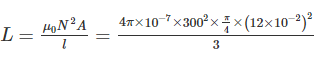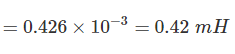QUESTION: 17

Midway between two equal and similar charges, a third equal and similar charge is placed. Then this third charge will ________.

Solution:

Midway between two equal and similar charges, a third equal and similar charge is placed. As the charge is placed midway between two similar charges, the force acts on the third charge due to two charges will be same. Hence third charge will remain in stable equilibrium.

QUESTION: 18

Which law states that “the line integral of the magnetic field intensity H around a closed path is equal to the total current linked by the contour”?

Solution:

The given statement is, the line integral of the magnetic field intensity H around a closed path is equal to the total current linked by the contour. Ampere’s circuit law states that the line integral of the magnetic field around some closed loop is equal to the times the algebraic sum of the currents which pass through the loop.

QUESTION: 19

A coil of 1000 turns is wound on a core. A current of 1 A flowing through the coil creates a core flux of 1 mWb. What is the energy stored in the magnetic field?

Solution: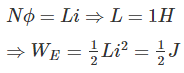QUESTION: 20

Which one of the following is the CORRECT value of magnetic permeability in free space?

Solution:

The factor µo is called the permeability of free space and has the value

4π × 10-7 newtons/ampere² in SI units. Since B is measured in tesla (newtons per ampere-meter), the SI units for H must therefore be amperes per meter.Use Code STAYHOME200 and get INR 200 additional OFF Use Coupon Code